Note: The other languages of the website are Google-translated. Back to English
Log inorRegisteror

## How to convert date to fiscal year/quarter/month in Excel?

If you have a list of dates in a worksheet, and you want to quickly confirm the fiscal year/quarter/month of these dates, you can read this tutorial, I think you may find the solution.

Convert date to fiscal year

Convert date to fiscal quarter

Convert date to fiscal month

####Convert date to fiscal year

1. Select a cell, and type the fiscal year starting month number into it, here, my company’s fiscal year starts from July 1st, and I type 7. See screenshot: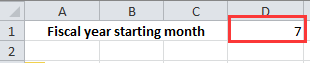2. Then you can type this formula =YEAR(DATE(YEAR(A4),MONTH(A4)+(\$D\$1-1),1)) into a cell next to your dates, then drag the fill handle to a range you need.Tip: In above formula, A4 indicates the date cell, and D1 indicates the month which the fiscal year starts in.

####Convert date to fiscal quarter

If you want to convert date to fiscal quarter, you can do as these:

1. Firstly, you need to make a table as below screenshot shown. In first row list all months of a year, then in the second row, type the relative fiscal quarter number to each month. See screenshot: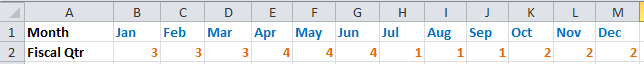2. Then in a cell next to your date column, and type this formula =CHOOSE(MONTH(A6),3,3,3,4,4,4,1,1,1,2,2,2) into it, then drag the fill handle to a range you need.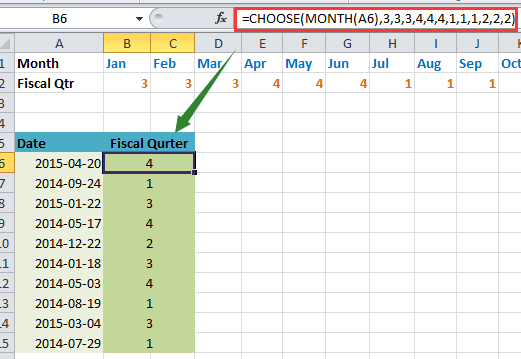Tip: In the above formula, A6 is the date cell, and the number series 3,3,3…is the fiscal quarter series you typed in step 1.

####Convert date to fiscal month

To convert date to fiscal month, you also need to make a table firstly.

1. In first row list all month of a year, then in the second row, type the relative fiscal month number to each month. See screenshot: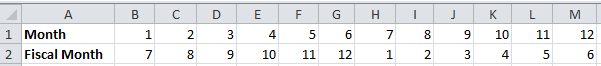2. Then in a cell next to the column, type this formula =CHOOSE(MONTH(A6),7,8,9,10,11,12,1,2,3,4,5,6) into it, and drag the fill handle to your needed range with this formula.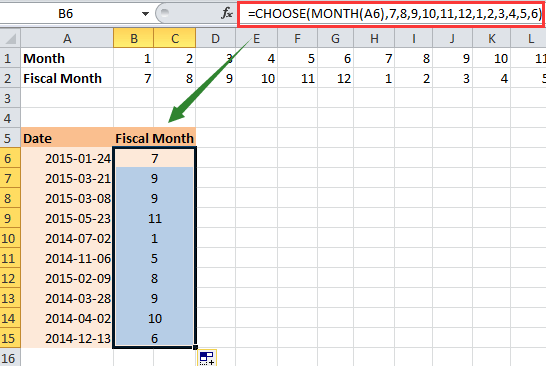Tip: In the above formula, A6 is the date cell, and the number series 7,8,9…is the fiscal month number series you type in step 1.

#### Quickly convert nonstandard date to standard date formattiing(mm/dd/yyyy)

In some times, you may received a workhseets with multiple nonstandard dates, and to convert all of them to the standard date formatting as mm/dd/yyyy maybe troublesome for you. Here Kutools for Excel's Conver to Date can quickly convert these nonstandard dates to the standard date formatting with one click.  Click for free full featured trial in 30 days!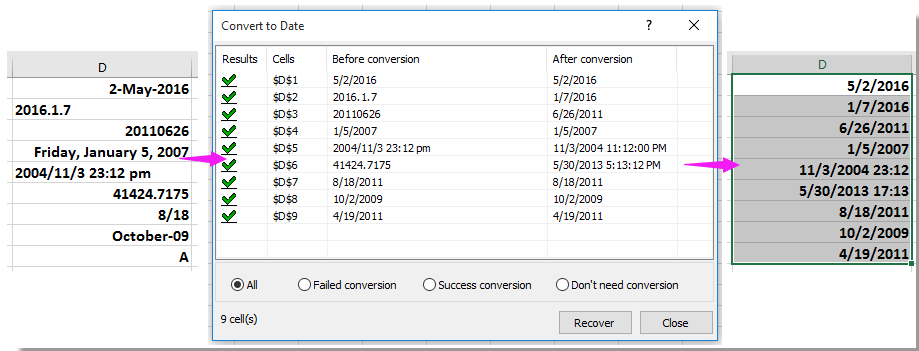### The Best Office Productivity Tools

#### Kutools for Excel Solves Most of Your Problems, and Increases Your Productivity by 80%

• Reuse: Quickly insert complex formulas, charts and anything that you have used before; Encrypt Cells with password; Create Mailing List and send emails...
• Super Formula Bar (easily edit multiple lines of text and formula); Reading Layout (easily read and edit large numbers of cells); Paste to Filtered Range...
• Merge Cells/Rows/Columns without losing Data; Split Cells Content; Combine Duplicate Rows/Columns... Prevent Duplicate Cells; Compare Ranges...
• Select Duplicate or Unique Rows; Select Blank Rows (all cells are empty); Super Find and Fuzzy Find in Many Workbooks; Random Select...
• Exact Copy Multiple Cells without changing formula reference; Auto Create References to Multiple Sheets; Insert Bullets, Check Boxes and more...
• Extract Text, Add Text, Remove by Position, Remove Space; Create and Print Paging Subtotals; Convert Between Cells Content and Comments...
• Super Filter (save and apply filter schemes to other sheets); Advanced Sort by month/week/day, frequency and more; Special Filter by bold, italic...
• Combine Workbooks and WorkSheets; Merge Tables based on key columns; Split Data into Multiple Sheets; Batch Convert xls, xlsx and PDF...
• More than 300 powerful features. Supports Office/Excel 2007-2019 and 365. Supports all languages. Easy deploying in your enterprise or organization. Full features 30-day free trial. 60-day money back guarantee.#### Office Tab Brings Tabbed interface to Office, and Make Your Work Much Easier

• Enable tabbed editing and reading in Word, Excel, PowerPoint, Publisher, Access, Visio and Project.
• Open and create multiple documents in new tabs of the same window, rather than in new windows.
• Increases your productivity by 50%, and reduces hundreds of mouse clicks for you every day!Say something here...
symbols left.
###### or post as a guest, but your post won't be published automatically.
• To post as a guest, your comment is unpublished.
· 27 days ago
Just check this

=IF(MONTH(I8)>3,"FY"&YEAR(I8)&"-"&YEAR(I8)+1,IF(MONTH(I8)<=3,"FY"&YEAR(I8)-1&"-"&YEAR(I8)))

Assume the target is in I8 cell with a date of 8/23/2011 , the out put will be FY2011-2012

Sathish
• To post as a guest, your comment is unpublished.
· 27 days ago
Just check this

=IF(MONTH(I8)>3,"FY"&YEAR(I8)&"-"&YEAR(I8)+1,IF(MONTH(I8)<=3,"FY"&YEAR(I8)-1&"-"&YEAR(I8)))

Assume the target is in I8 cell with a date of 8/23/2011 , the out put will be

Sathish
• To post as a guest, your comment is unpublished.
· 1 years ago
If anyone is looking for a formula to figure out what fiscal/policy year an payment/event happened, even if that fiscal year/policy doesn't start on the first of the month I came up with this.

=YEAR(A4)+((MONTH(A4)>=MONTH(\$D\$1)+(DAY(A4)<Day(\$D\$1)))-1)

D1 in this has to be a full date 01/06/2019 for example.

Also surely a cheeky Vlookup is a better way of doing the quarter formula

=Vlookup(Month(A6),etc.)
• To post as a guest, your comment is unpublished.
· 1 years ago
I came up with a self contained choose() to calculate the fiscal year that doesn't need to use an external cell as an input.
.
Fiscal Year EndCalculation
="June 30, " & (YEAR(E2) +CHOOSE(MONTH(E2),0,0,0,0,0,0,1,1,1,1,1,1))
. * The first part is just a text string
. * & concatenates the first part to the second part which calculatesthe appropriate year.
. * Year(e2) extracts the year number from the date in column E
. * Choose() looks at the month number from that same date.
. For months Jan through June itretrieves the value 0,
. For months July thru Dec itretrieves value 1.
. The retrieved value is added to theyear extracted to give the appropriate year end
“June 30, 2020”.

This is on the assumption that a company is not likely to be changing it's year end. Doesn't work if the sheet is being used for multiple companies with different year ends ...
• To post as a guest, your comment is unpublished.
· 2 years ago
Please correct the fiscal-year formula you give above! It does not work unless the fiscal year begins with month 7. This formula will work just fine:

=YEAR(DATE(YEAR(A4),MONTH(A4)+12-(\$D\$1-1),1))

But it is still way more complex than it needs to be. Use Michael's formula (but correct his typo):

=YEAR(A4)+(MONTH(A4)>=\$D\$1)
• To post as a guest, your comment is unpublished.
· 2 years ago
IF YOU WANT TO FIND FISCAL YEAR IN INDIA....
WRITE EXCEL QUERY AS BELOW

=(YEAR(DATE)+(MONTH(DATE)>=4))-1
• To post as a guest, your comment is unpublished.
· 3 years ago
You can use below formulae:

Here Col K2 is our date column which is to be converted in format 20XX-XY (e.g. 2017-18).

=IF(MONTH(K2)<4,TEXT(DATE(YEAR(K2)-1,MONTH(K2),DAY(K2)),"YYYY-")&TEXT(DATE(YEAR(K2),MONTH(K2),DAY(K2)),"YY"),TEXT(DATE(YEAR(K2),MONTH(K2),DAY(K2)),"YYYY-")&TEXT(DATE(YEAR(K2)+1,MONTH(K2),DAY(K2)),"YY"))
• To post as a guest, your comment is unpublished.
· 2 years ago
Thank you!!
• To post as a guest, your comment is unpublished.
· 2 years ago
What a legend. Thank you!
• To post as a guest, your comment is unpublished.
· 2 years ago
thank you
• To post as a guest, your comment is unpublished.
· 3 years ago
To calculate the FISCAL YEAR, MONTH, and QUARTER of a given date (here in A4), these formulas will work for any FY starting month (here in \$D\$1):
FISCAL YEAR =YEAR(A4)+(MONTH(A4)]=\$D\$1)
FISCAL MONTH =MOD(MONTH(A4)-\$D\$1;12)+1
FISCAL QUARTER =QUOTIENT(MOD(MONTH(A4)-\$D\$1;12);3)+1

How it works (examples assume FY starting month in \$D\$1 = July = 7):

As in MS EXCEL the equivalent value for TRUE = 1 and FALSE = 0, the FISCAL YEAR can also be calculated without the IF clause as:
FISCAL YEAR =YEAR(A4)+(MONTH(A4)]=\$D\$1)

B. In the Fiscal Month formula, the innermost term [MONTH(A4) - \$D\$1] will shift the months by the FY starting month, i.e. JUL(7) - StartMonth(7) = 7 - 7 = 0.
So the result for date in fiscal start month = 0, while all others range from -11..+11, e.g. Jul = 0, Aug= 1, ... , Dec = 5; Jan = -6; Feb = -5; Jun = -1

C. Running the result [B] above through the term [MOD([B];12)], will retain the positive numbers, while increasing any negative [B] by 12.
Now the results range from 0.11, e.g. Jul = 0, Aug= 1, ... , Dec = 5; Jan = 6; Feb = 7; Jun = 11

D. The final [+1] term will adjust the 0..11 range of [C] to be the correct 1..12 fiscal month range, so the FiscalStartMonth = 1, et voila:
e.g. Jul = 1, Aug= 2, ... , Dec = 6; Jan = 7; Feb = 8; Jun = 12

E. Converting a FISCAL MONTH to a FISCAL QUARTER becomes relatively easy then, knowing that quarter (= 3 months) can be calculated by
QUARTER = QUOTIENT( [Month] - 1 ; 3) + 1

F. Replacing [Month] with [Fiscal Month] in above formula to get .......... QUOTIENT( [Fiscal Month] - 1 ; 3) + 1
Now we can insert the FISCAL MONTH formula from above and get .... QUOTIENT( MOD(MONTH(A4)-\$D\$1;12)+1 - 1 ; 3) + 1
The [+1 -1] in the middle can be removed, and we end up with ............. QUOTIENT( MOD(MONTH(A4)-\$D\$1;12) ; 3) + 1
• To post as a guest, your comment is unpublished.
· 3 years ago
In the Fiscal Year example, the formula in cell D4 should be:
=YEAR(A4)+IF(MONTH(A4)>=\$D\$1,1,0)
This will work for any fiscal year, not just one that starts with July
• To post as a guest, your comment is unpublished.
· 4 years ago
Hi

Our fiscal year starts in February and ends in January of the following year (for example 1-Feb-2017 to 31-Jan-2018 should = Fiscal Year 2018, hence 31-Jul-2017 should be in Fiscal Year 2018, your formula computes Fiscal Year 2017). So I did everything as you stated above and changed the "Fiscal year starting month" to 2 and it does not calculate it right for some dates. I am not sure why. I would appreciate if you could please get back to me. Many thanks
• To post as a guest, your comment is unpublished.
· 4 years ago
[quote name="Edward"]Hi

Our fiscal year starts in February and ends in January of the following year (for example 1-Feb-2017 to 31-Jan-2018 should = Fiscal Year 2018, hence 31-Jul-2017 should be in Fiscal Year 2018, your formula computes Fiscal Year 2017). So I did everything as you stated above and changed the "Fiscal year starting month" to 2 and it does not calculate it right for some dates. I am not sure why. I would appreciate if you could please get back to me. Many thanks[/quote]

Would this work for you?

=IF(MONTH(A1)>=2,YEAR(A1)+1,YEAR(A1))
Any month >=Feb would return the current year+1 which is your FY. Otherwise, it will only return the year as is. When I did Ctrl+: in cell A1 to paste date in MS Excel, it returned 2018. Changed the 04 to a 01 and it returned 2017.
• To post as a guest, your comment is unpublished.
· 3 years ago
WOW so easy thanks!!
• To post as a guest, your comment is unpublished.
· 4 years ago
Would it be easier to do this [b]MONTH(A4)+(6)[/b] instead of [b]MONTH(A4)+(\$D\$1-1)[/b] for the fiscal year calculations?

Thanks for the post!
• To post as a guest, your comment is unpublished.
· 4 years ago
An easy formula for Fiscal Month:
=IF(+TEXT(Index!E2,"M")-6
• To post as a guest, your comment is unpublished.
· 4 years ago